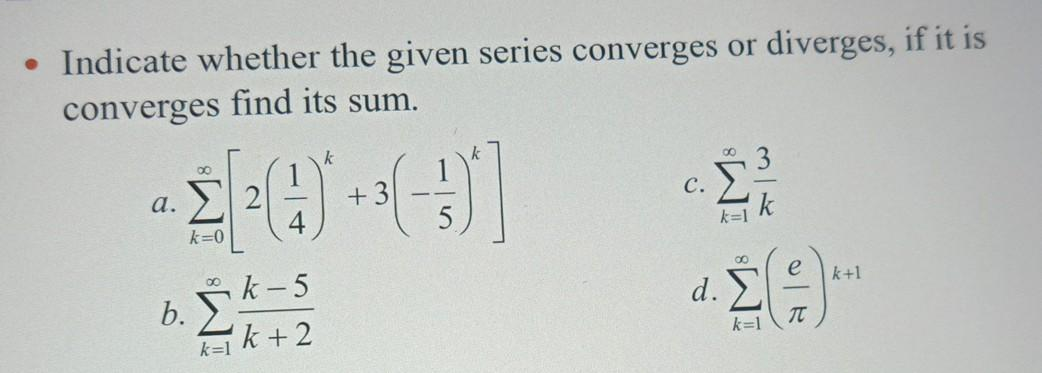### Create an Account

Home / Questions / • Indicate whether the given series converges or diverges, if it is converges find its sum...

# • Indicate whether the given series converges or diverges, if it is converges find its sum. O 3 C. Σ α. Σ 2 Σ * e k+1 k - 5 d. b. Σ k=1 π kek +2

• Indicate whether the given series converges or diverges, if it is converges find its sum. O 3 C. Σ α. Σ 2 Σ * e k+1 k - 5 d. b. Σ k=1 π kek +2Apr 15 2021 View more View LessSubscribe To Get Solution